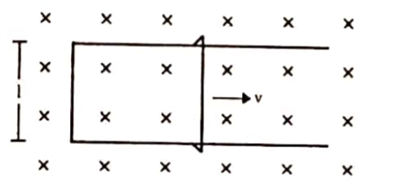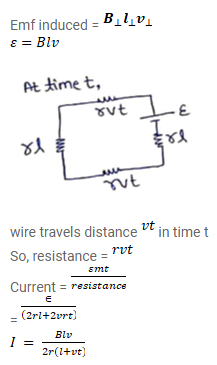# Figure shows a long U-shaped wire of length I placed in a perpendicular magnetic field

Question:

Figure shows a long U-shaped wire of length I placed in a perpendicular magnetic field B. A wire of length I is slid on the Ushaped wire with a constant velocity $v$ towards right. The resistance of all the wires is r per unit length. At $t=0$, the sliding wire is close to the left edge of the U-shaped wire. Draw an equivalent circuit diagram, showing the induced emf as a battery. Calculate the current in the circuit.Solution: